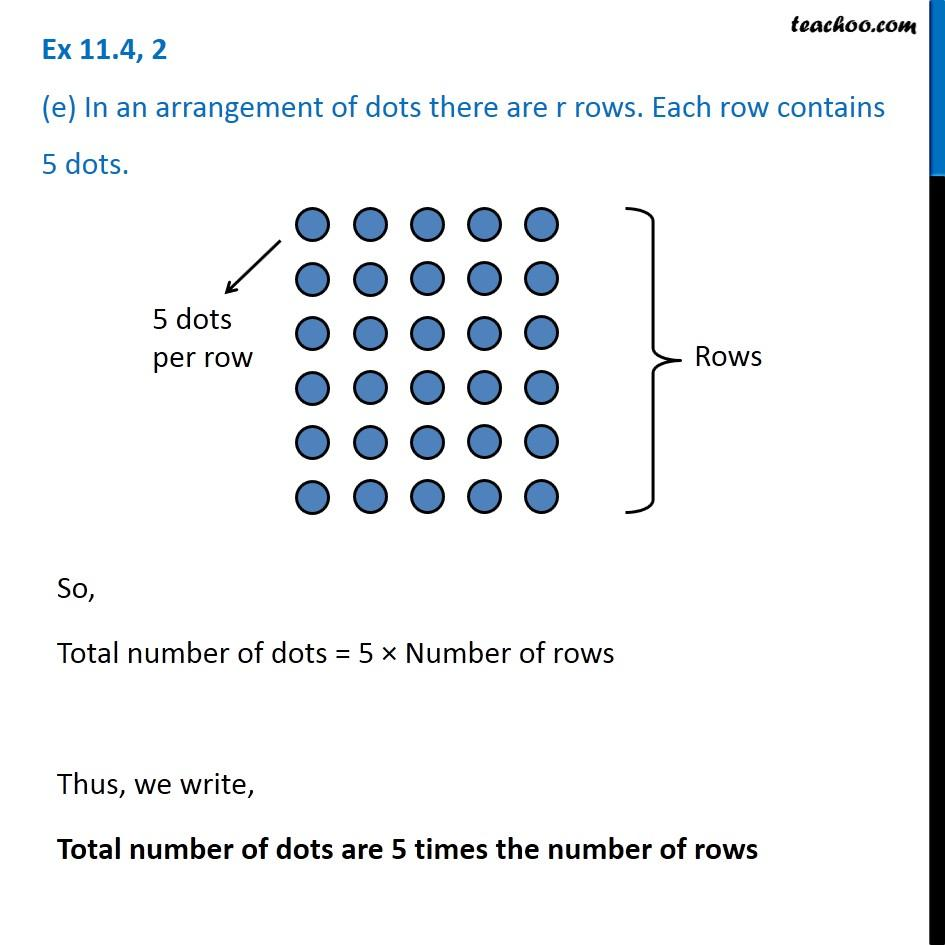Creating Algebra Expressions from Word Problems - Worksheet

Chapter 11 Class 6 Algebra
Serial order wiseLearn in your speed, with individual attention - Teachoo Maths 1-on-1 Class

### Transcript

Question 2 (e) In an arrangement of dots there are r rows. Each row contains 5 dots.So, Total number of dots = 5 × Number of rows Thus, we write, Total number of dots are 5 times the number of rows